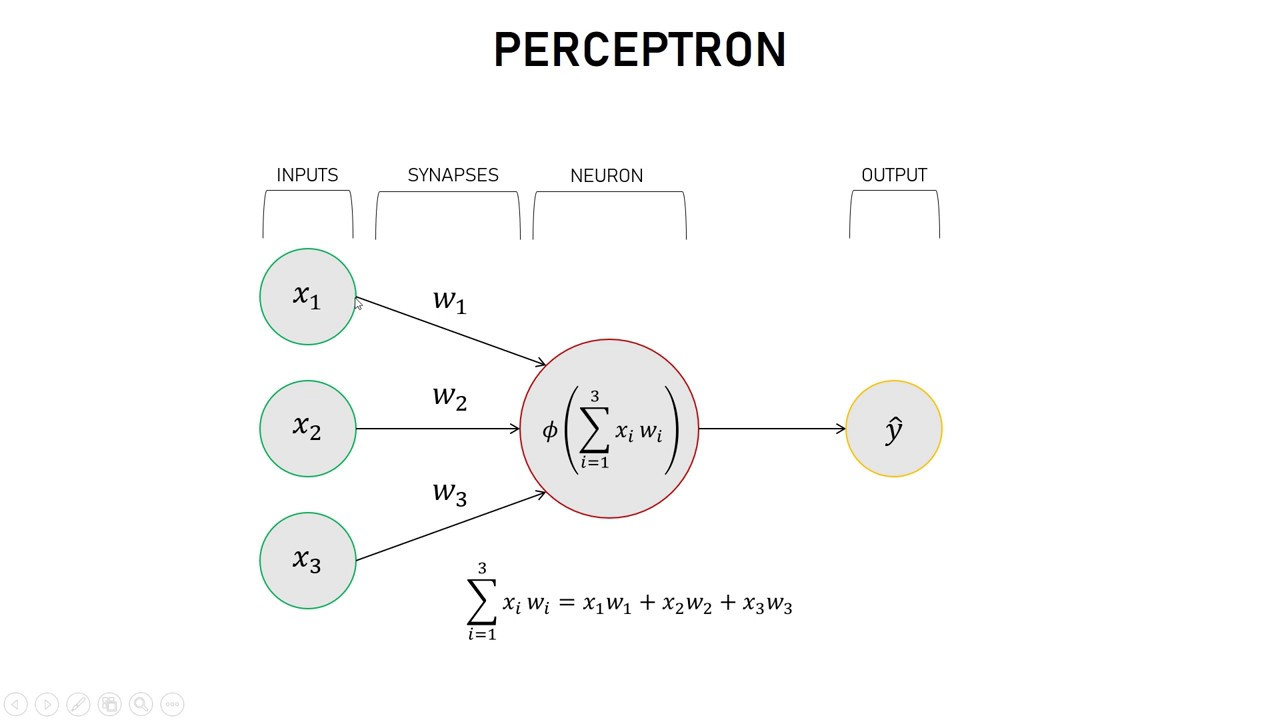1559528449

# Create a Simple Neural Network in Python from Scratch

In this video I’ll show you how an artificial neural network works, and how to make one yourself in Python. In the next video we’ll make one that is usable, but if you want, that code can already be found on github. I recommend watching at 1.5x speed, unless you’re coding along.

Github code for complete neural network:

https://github.com/jonasbostoen/simple-neural-network

### Create a Simple Neural Network in Python from Scratch - Part 2

#python #data-science #deep-learning

## Buddha Community1619518440

## top 30 Python Tips and Tricks for Beginners

Welcome to my Blog , In this article, you are going to learn the top 10 python tips and tricks.

### 8) Check The Memory Usage Of An Object.

#python #python hacks tricks #python learning tips #python programming tricks #python tips #python tips and tricks #python tips and tricks advanced #python tips and tricks for beginners #python tips tricks and techniques #python tutorial #tips and tricks in python #tips to learn python #top 30 python tips and tricks for beginners1619510796

## Lambda, Map, Filter functions in python

Welcome to my Blog, In this article, we will learn python lambda function, Map function, and filter function.

Lambda function in python: Lambda is a one line anonymous function and lambda takes any number of arguments but can only have one expression and python lambda syntax is

Syntax: x = lambda arguments : expression

Now i will show you some python lambda function examples:

#python #anonymous function python #filter function in python #lambda #lambda python 3 #map python #python filter #python filter lambda #python lambda #python lambda examples #python map1624752180

## How to build your own Neural Network from scratch in Python

When discussing neural networks, most beginning textbooks create brain analogies. I can define the new neural networks simply as a mathematical function that translates a certain entry to the desired performance without going into brain analogies.

You may note that the weights W and biases b are the only variables in the equation above affecting the output of a given value. The strength of predictions naturally establishes the correct values for weights and biases. The weight and bias adjustment procedure of the input data is known as neural network training.

#neural-networks #artificial-intelligence #python #programming #technology #how to build your own neural network from scratch in python1623135499

## No Code introduction to Neural Networks

### The simple architecture explained

Neural networks have been around for a long time, being developed in the 1960s as a way to simulate neural activity for the development of artificial intelligence systems. However, since then they have developed into a useful analytical tool often used in replace of, or in conjunction with, standard statistical models such as regression or classification as they can be used to predict or more a specific output. The main difference, and advantage, in this regard is that neural networks make no initial assumptions as to the form of the relationship or distribution that underlies the data, meaning they can be more flexible and capture non-standard and non-linear relationships between input and output variables, making them incredibly valuable in todays data rich environment.

In this sense, their use has took over the past decade or so, with the fall in costs and increase in ability of general computing power, the rise of large datasets allowing these models to be trained, and the development of frameworks such as TensforFlow and Keras that have allowed people with sufficient hardware (in some cases this is no longer even an requirement through cloud computing), the correct data and an understanding of a given coding language to implement them. This article therefore seeks to be provide a no code introduction to their architecture and how they work so that their implementation and benefits can be better understood.

Firstly, the way these models work is that there is an input layer, one or more hidden layers and an output layer, each of which are connected by layers of synaptic weights¹. The input layer (X) is used to take in scaled values of the input, usually within a standardised range of 0–1. The hidden layers (Z) are then used to define the relationship between the input and output using weights and activation functions. The output layer (Y) then transforms the results from the hidden layers into the predicted values, often also scaled to be within 0–1. The synaptic weights (W) connecting these layers are used in model training to determine the weights assigned to each input and prediction in order to get the best model fit. Visually, this is represented as:

#machine-learning #python #neural-networks #tensorflow #neural-network-algorithm #no code introduction to neural networks1602968400

## Python Tricks Every Developer Should Know

Python is awesome, it’s one of the easiest languages with simple and intuitive syntax but wait, have you ever thought that there might ways to write your python code simpler?

In this tutorial, you’re going to learn a variety of Python tricks that you can use to write your Python code in a more readable and efficient way like a pro.

### Let’s get started

Swapping value in Python

Instead of creating a temporary variable to hold the value of the one while swapping, you can do this instead

``````>>> FirstName = "kalebu"
>>> LastName = "Jordan"
>>> FirstName, LastName = LastName, FirstName
>>> print(FirstName, LastName)
('Jordan', 'kalebu')
``````

#python #python-programming #python3 #python-tutorials #learn-python #python-tips #python-skills #python-development